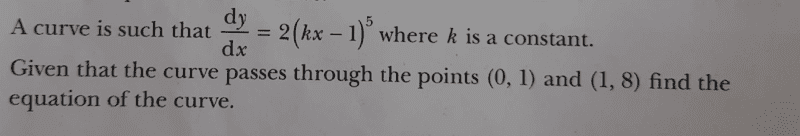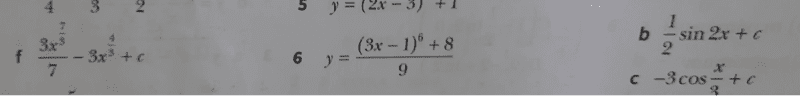# Find the equation of the curve given: ##\frac{dy}{dx}=2(kx-1)^5##

• chwala
Whatever we do, we will have the equation ##21k=(k-1)^6-1.## I did another substitution, ##s:=k-1,## got ##s^6-21s-22=0## where you can read of ##s=-1## immediately. I asked WA for the solution, but you can of course do the long division ##(s^6-21s-22):(s+1)=-22 + s - s^2 + s^3 - s^4 + s^5## and see whether you can guess ##s=2,## or check ##\pm1, \pm 2, \pm 11## as divisors of ##22##f

#### chwala

Gold Member
Homework Statement
See attached textbook question and solution
Relevant Equations
Integration - Calculus
This is the question...hmmmm it stressed me a little bit.Find the textbook solution here; no. 6Now my approach to this was as follows;

On integration,

##y=\dfrac{(kx-1)^6}{3k} +c##

on using the point ##(0,1)## and ##(1,8)##, we end up with

##1=\dfrac{1}{3k} +c##
##8=\dfrac{(k-1)^6}{3k} +c##

it follows that,

##8=\dfrac{(k-1)^6}{3k} + \dfrac{3k-1}{3k} ##
...
##(k-1)^6+3k-1-24k=0##

##(k-1)^6-21k-1=0##

##k^6-6k^5+15k^4-20k^3+15k^2-6k+1-21k-1=0##

I let ##P(k)=k^6-6k^5+15k^4-20k^3+15k^2-6k+1-21k-1##

using trial and error method from ##±0, ±1...## i got ##P(3)=0## implying ##k=3## is a factor,

thus substituting on;

##1=\dfrac{1}{3k} +c##

we get;

##1=\dfrac{1}{3×3} +c##

##1=\dfrac{1}{9} +c##

##c=\dfrac{8}{9}##

Therefore;

##y=\dfrac{(3x-1)^6}{3×3} +\dfrac{8}{9}##

##y=\dfrac{(3x-1)^6+8}{9}##

I would be interested if there would be another approach to this question. Cheers guys.

Last edited:
Homework Statement:: See attached textbook question and solution
Relevant Equations:: Integration - Calculus

I would be interested if there would be another approach to this question. Cheers guys.
With ##t:=kx-1## and integration you get quickly to ##y=\dfrac{2}{6k}(kx-1)^6+c## with two equations and two unknowns.

With ##t:=kx-1## and integration you get quickly to ##y=\dfrac{2}{6k}(kx-1)^6+c## with two equations and two unknowns.
Hi @fresh_42 ...that is the same approach that i used. Maybe i ought to be specific...is there a different way other than using the binomial expansion?

Hi @fresh_42 ...that is the same approach that i used. Maybe i ought to be specific...is there a different way other than using the binomial expansion?
Whatever we do, we will have the equation ##21k=(k-1)^6-1.## I did another substitution, ##s:=k-1,## got ##s^6-21s-22=0## where you can read of ##s=-1## immediately. I asked WA for the solution, but you can of course do the long division ##(s^6-21s-22):(s+1)=-22 + s - s^2 + s^3 - s^4 + s^5## and see whether you can guess ##s=2,## or check ##\pm1, \pm 2, \pm 11## as divisors of ##22## and hope that the solution is an integer solution.

At least it does not use binomial expansion, only division. But you cannot make the polynomial go away.

•chwala